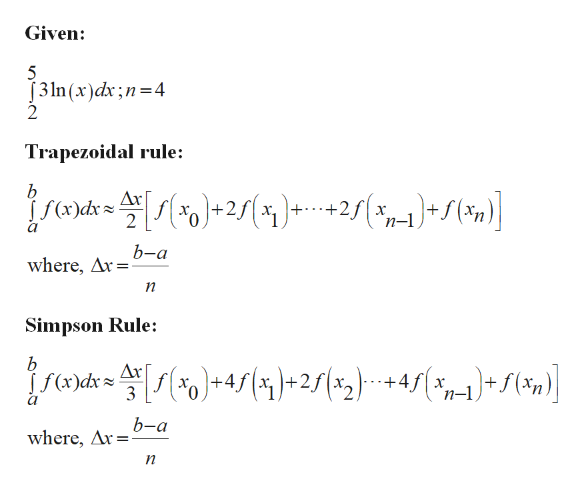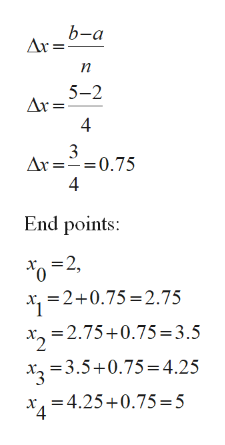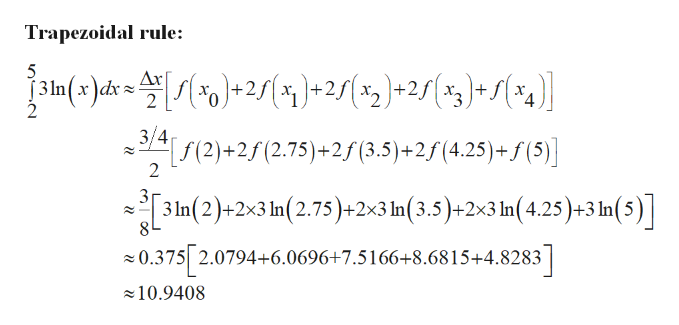# Use the trapezoidal rule and Simpson's rule to approximate the value of the definite integral. Compare your result with the exact value of the integral. (Give your answers correct to 4 decimal places.) 2∫53ln(x)dx; n=4

Question

Use the trapezoidal rule and Simpson's rule to approximate the value of the definite integral. Compare your result with the exact value of the integral. (Give your answers correct to 4 decimal places.)

253ln(x)dx; n=4

check_circle

Step 1

Evaluate the definite integral using trapezoidal rule and Simpson’s rule. And compare with exact value.help_outlineImage TranscriptioncloseGiven: 5 J3ln (x)dx;n 4 Trapezoidal rule: b Дх Хо 2 n-1 b-a where, Ar Simpson Rule: b Дк )-re [s(*)*+4s {y)+251x2) +4s( f(x)dx 3 n-1 b-a where, Ar n fullscreen
Step 2

From the given information, a= 2, b= 5 and n=4.help_outlineImage Transcriptioncloseb-а п 5-2 Ar = 4 3 Ax = 0.75 4 End points: -2, =2+0.75 2.75 xz=2.75+0.75 =3.5 =3.5+0.75 4.25 -4.25+0.75 5 4 fullscreen
Step 3

Substitute known...help_outlineImage TranscriptioncloseTrapezoidal rule: 5 In(x)dk = 2)-2s(%2)+25(ag)* S(%g)] (2)+2 (2.75)+ 2f (3.5) +2f (4.25)+f (5) 2 31n(2)+2x3 n(2.75)+2x3 In(3.5)+2x3 In (4,25)+3 In(5)] 8 0.375 2.0794+6.0696+7.5166+8.6815+4.8283 10.9408 fullscreen

### Want to see the full answer?

See Solution

#### Want to see this answer and more?

Solutions are written by subject experts who are available 24/7. Questions are typically answered within 1 hour.*

See Solution
*Response times may vary by subject and question.
Tagged in

### Integration